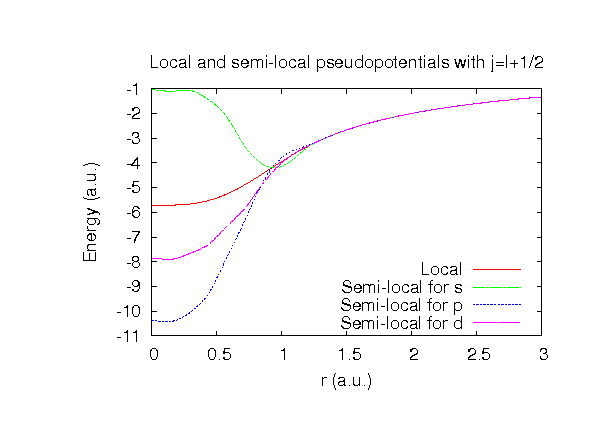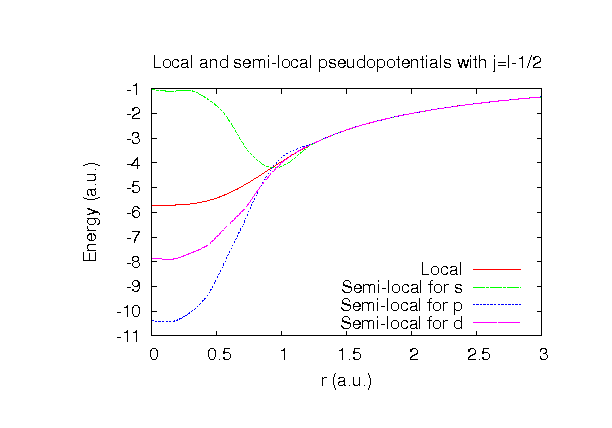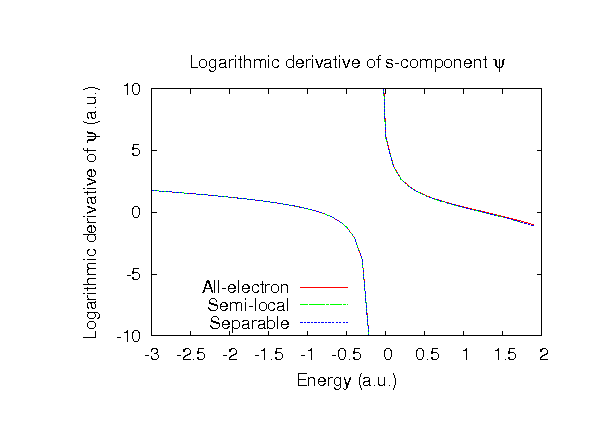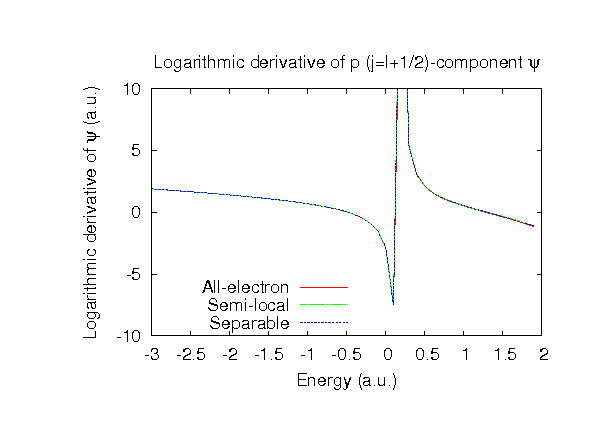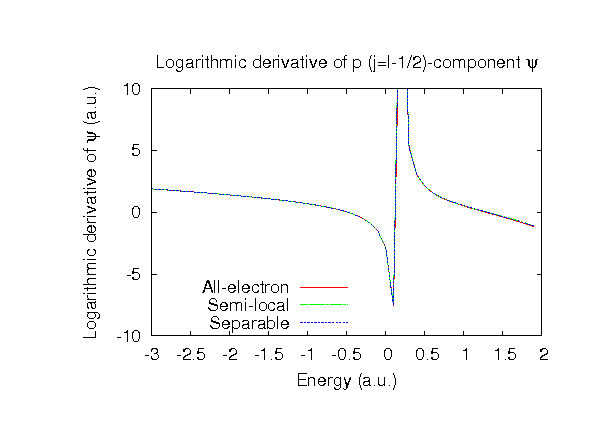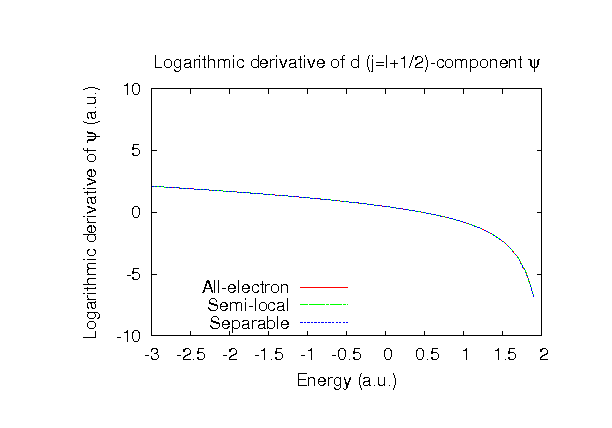### Fully relativistic pseudopotentials

Fully relativistic pseudopotentials generated by the MBK (PRB 47, 6728 (1993)) scheme within LDA (CA13) and GGA (PBE13) which contain a partial core correction and fully relativistic effects including spin-orbit coupling.

### Pseudo-atomic basis functions

The number below the symbol means a cutoff radius (a.u.) of the confinement potential. These file includes fifteen radial parts for each angular momentum quantum number l (=0,1,2,3). The basis functions were generated by variationally optimizing the corresponding primitive basis functions in the single atom and the dimer molecule. The input files used for the orbital optimization can be found at C_opt.dat and C2_opt.dat . Since C_CA13.vps and C_PBE13.vps include the 2s and 2p states (4 electrons) as the valence states, the minimal basis set is C*.*-s1p1. Our recommendation for the choice of cutoff radius of basis functions is that C5.0.pao is enough for bulks, but C6.0.pao or C7.0.pao is preferable for molecular systems.

### Benchmark calculations by the PBE13 pseudopotential with the various basis functions

(1) Calculation of the total energy as a function of lattice constant in the diamond structure, where the total energy is plotted relative to the minimum energy for each case. a0 and B0 are the equilibrium lattice constant and bulk modulus obtained by fitting to the Murnaghan equation of state. The difference between C6.0-s2p2d1 and C6.0-s3p3d2 in the total energy at the minimum point is 0.026 eV/atom. An input file used for the OpenMX calculations can be found at Cdia-EvsV.dat . For comparison the result by the Wien2k code is also shown, where the calculation was performed by default setting in the Ver. 10.1 of Wien2k.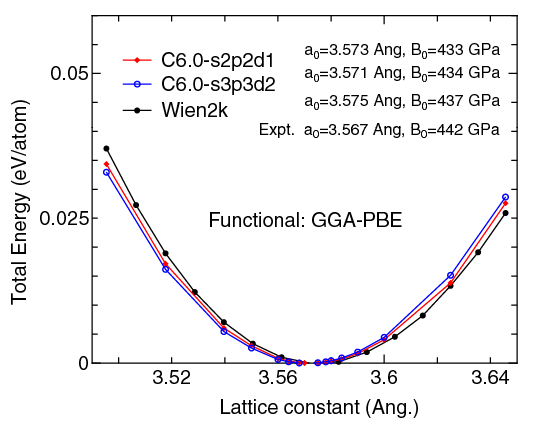(2) Calculation of the band dispersion in the diamond structure, where the non-spin polarized collinear calculation with the lattice constant of 3.567 Ang. was performed using C_PBE13.vps and C6.0-s2p2d1, and the Fermi level is taken to be the top of valence band. The input file used for the OpenMX calculations can be found at Cdia-Band.dat . For comparison the result by the Wien2k code is also shown, where the calculation was performed by default setting in the Ver. 10.1 of Wien2k.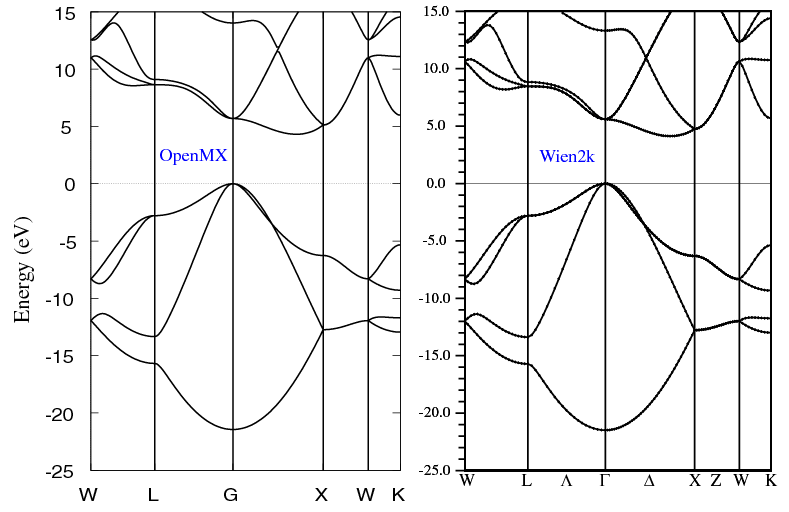(3) Calculations of a C2H2 molecule, where C_PBE13.vps, O_PBE13.vps, C7.0-s2p2d1, O7.0-s2p2d1, C7.0-s3p3d2, and O7.0-s3p3d2 were used. The input files used for the OpenMX calculations can be found at C2H2.dat , H_C2H2_1.dat , C_C2H2_1.dat , H_C2H2_2.dat , C_C2H2_2.dat , H_C2H2_cp1.dat , C_C2H2_cp1.dat , H_C2H2_cp2.dat , and C_C2H2_cp2.dat .

 Equilibrium C-C bond length (Ang.) Equilibrium C-H bond length (Ang.) Atomization energy (kcal/mol) Atomization energy (couterpoise corrected) (kcal/mol) C7.0-s2p2d1, H7.0-s2p1 1.216 1.075 410.9 409.7 C7.0-s3p3d2, H7.0-s3p2 1.212 1.071 414.6 413.2 Other calc. 1.226 a 1.087 a 414.9 b Expt. 1.203c 1.063c 405.4b

a M. Krack, Theor. Chem. Acc. 114, 145 (2005).
b S. Kurth, J.P. Perdew, and P. Blaha, Int. J. Quan. Chem. 75, 889 (1999).
c G. Graner, E. Hirota, T. Iijima, K. Kuchitsu, D.A. Ramsay, J. Vogt, N. Vogt, Structure data of free polyatomic molecules. In: Kuchitsu K (ed) Landolt-Bornstein, New series, Group II: molecules and radicals, vol 25. Springer, Berlin Heidelberg NewYork.

### Supplementary information for the GGA (PBE13) pseudopotential